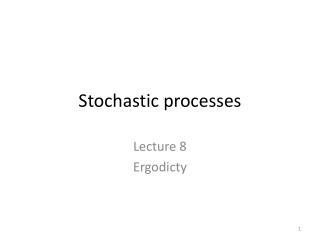DownloadDownload PresentationS tochastic processes

# S tochastic processes

Télécharger la présentation## S tochastic processes

- - - - - - - - - - - - - - - - - - - - - - - - - - - E N D - - - - - - - - - - - - - - - - - - - - - - - - - - -
##### Presentation Transcript

1. Stochastic processes Lecture 8 Ergodicty

2. Random process

3. Agenda (Lec. 8) • Ergodicity • Central equations • Biomedical engineering example: • Analysis of heart sound murmurs

4. Ergodicity • A random process X(t) is ergodic if all of its statistics can be determined from a sample function of the process • That is, the ensemble averages equal the corresponding time averages with probability one.

5. Ergodicity ilustrated • statistics can be determined by time averaging of one realization

6. Ergodicity and stationarity • Wide-sense stationary: Mean and Autocorrelation is constant over time • Strictly stationary: All statistics is constant over time

7. Weak forms of ergodicity • The complete statistics is often difficult to estimate so we are often only interested in: • Ergodicity in the Mean • Ergodicity in the Autocorrelation

8. Ergodicity in the Mean • A random process is ergodic in mean if E(X(t)) equals the time average of sample function (Realization) • Where the <> denotes time averaging • Necessary and sufficient condition: X(t+τ) and X(t) must become independent as τ approaches ∞

9. Example • Ergodic in mean: X • Where: • is a random variable • a and θ are constant variables • Mean is impendent on the random variable • Not Ergodic in mean: X • Where: • and dcr are random variables • a and θ are constant variables • Mean is not impendent on the random variable

10. Ergodicity in the Autocorrelation • Ergodic in the autocorrelation mean that the autocorrelation can be found by time averaging a single realization • Where • Necessary and sufficient condition: X(t+τ) X(t)and X(t+τ+a) X(t+a) must become independent as a approaches∞

11. Example (1/2)Autocorrelation • A random process • where A and fc are constants, and Θ is a random variable uniformly distributed over the interval [0, 2π] • The Autocorraltion of of X(t) is: • What is the autocorrelation of a sample function?

12. Example (2/2) • The time averaged autocorrelation of the sample function Thereby

13. Ergodicity of the First-Order Distribution • If an process is ergodic the first-Order Distribution can be determined by inputting x(t) in a system Y(t) • And the integrating the system • Necessary and sufficient condition: X(t+τ) and X(t) must become independent as τ approaches ∞

14. Ergodicity of Power Spectral Density • A wide-sense stationary process X(t) is ergodic in power spectral density if, for any sample function x(t),

15. Example • Ergodic in PSD: X • Where: • is a random variable • a and are constant variables • The PSD is impendent on the phase the random variable • Not Ergodic in PSD: X • Where: • are random variables • a and θ are constant variables • The PSD is not impendent on the random variable

16. Essential equations

17. Typical signals • Dirac delta δ(t) • Complex exponential functions

18. Essential equationsDistribution and density functions First-order distribution: First-order density function: 2end order distribution 2end order density function

19. Essential equations Expected value 1st order (Mean) • Expected value (Mean) • In the case of WSS • In the case of ergodicity Where<> denotes time averaging such as

20. Essential equations Auto-correlations • In the general case • Thereby • If X(t) is WSS • If X(i) is Ergodic • where

21. Essential equations Cross-correlations • In the general case • In the case of WSS

22. Properties of autocorrelation and crosscorrelation • Auto-correlation: Rxx(t1,t1)=E[|X(t)|2] When WSS: Rxx(0)=E[|X(t)|2]=σx2+mx2 • Cross-correlation: • If Y(t) and X(t) isindependent Rxy(t1,t2)=E[X(t)Y(t)]=E[X(t)]E[Y(t)] • If Y(t) and X(t) is orthogonal Rxy(t1,t2)=E[X(t)Y(t)]=E[X(t)]E[Y(t)]=0;

23. Essential equationsPSD • Truncated Fourier transform of X(t): • Power spectrum • Or from the autocorrelation • The Fourier transform of the auto-correlation

24. Essential equationsLTI systems (1/4) • Convolution in time domain: Where h(t) is the impulse response Frequency domain: Where X(f) and H(f) is the Fourier transformed signal and impluse response

25. Essential equationsLTI systems (2/4) • Expected value (mean) of the output: • If WSS: • Expected Mean square value of the output • If WSS:

26. Essential equationsLTI systems (3/4) • Cross correlation function between input and output when WSS • Autocorrelation of the output when WSS

27. Essential equationsLTI systems (4/4) • PSD of the output • Where H(f) is the transfer function • Calculated as the four transform of the impulse response

28. A biomedical example on a stochastic process • Analyze of Heart murmurs from Aortic valve stenosis using methods from stochastic process.

29. Introduction to heart sounds • The main sounds is S1 and S2 • S1 the first heart sound • Closure of the AV valves • S2 the second heart sound • Closure of the semilunar valves

30. Aortic valve stenosis • Narrowing of the Aortic valve

31. Reflections of Aortic valve stenosis in the heart sound • A clear diastolic murmur which is due to post stenoticturbulence

32. Abnormal heart sounds

33. Signals analyze for algorithm specification • Is heart sound stationary, quasi-stationary or non-stationary? • What is the frequency characteristic of systolic Murmurs versus a normal systolic period?

34. exercise • Chi meditation and autonomic nervous system